— ART HISTORY & IMAGE STUDIES —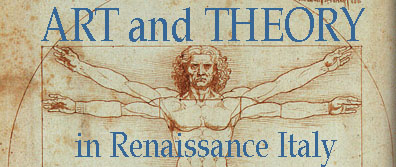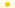ARTH 341SCHEDULEREQUIREMENTSREAD Alberti, On Painting, Book I, 54-59 Perspective ...construction of intersection in painting how to construct the intersection - how to express the intersection in painting. "First of all, on the the surface on which I am going to paint, I draw a rectangle of whatever size I want, which I regard as an open window through which the subject to be painted is seen; and I decide how large I wish the human figures in the painting to be"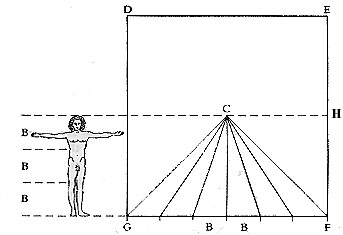Construction of perspective, first stage: 'parallel' lines drawn to the "centric point" C = "centric point" B = one braccio module (one-third the height of a man) The height of the man at the front plane of the picture gives the height of the horizon at H The braccio divisions along GF are joined to the perspective focus at C to give the orthogonals "I divide the height of man into three parts, which will be proportional to the measure commonly called a braccio [i.e. an arm's length]; for, as may be seen from the relationship of his limbs, three braccia is just about the average height of a man's body." (the braccio in Florence is equivalent to about 58 centimetres or 23 inches) "With this measure I divide the bottom line of my rectangle into as many parts as it will hold; and this bottom line of the rectangle is for me proportional to the nearest transverse equidistant seen on the pavement. "Then I establish a point in the rectangle wherever I wish; and as it occupies the place where the centric ray strikes, I shall call it the centric point." "The suitable position for this centric point is no higher from the base line than the height of the man to be represented in the painting, for in this way both the viewers and the objects in the painting will be seen on the same plane." "Having placed the centric point, I draw straight lines from it to each of the divisions on the base line. These lines show me how successive transverse quantities visually change to an almost infinite distance." "At this stage some would draw a line across the rectangle equidistant from the divided line [the 'horizon line'], and then divide the space between these two lines into three parts" - see Fig. 9. [lines MN and OP] "Then to that second equidistant line [OP] they would add another above [QR], following the rule that the space which is divided into three parts between the first divided (base) line and the second equidistant one, shall exceed by one of its parts the space between the second and third lines...[and so on] "That would be their way of proceeding..." - and leads to "quite serious mistakes" in painting "...I discovered the following excellent method." [same as above] "But as regards the successive transverse quantities I observe the following method." "I have a drawing surface on which I describe a single straight line, and this I divide into parts like those into which the base line of the rectangle is divided"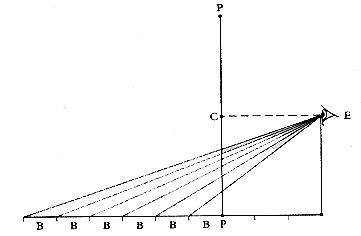Construction in perspective, second stage determination of the horizontal divisions on the intersection "Then I place a point above this line, directly over one end of it, at the same height as the centric point is from the base line of the rectangle, and from this point I draw lines to each of the divisions on the line." "Then I determine the distance I want between the eye of the spectator and the painting, and, having established the position of the intersection at this distance, I effect the intersection with what mathematicians call a perpendicular." "A perpendicular is a line which at the intersection with another straight line makes right angles on all sides." where the perpendicular intersects the lines coming from the base line indicates the distance between the "transverse equidistant lines of the pavement.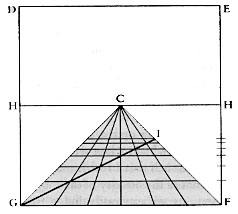Construction in perspective, third stage completion of the squared "pavement" DEFG The intervals on the intersection PP identified in the second stage (see above) are transferred to HF (or HG) and the successive horizontals of the "pavement" are drawn at corresponding levels GI drawn as proof of the accuracy of the construction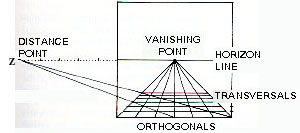Construction in perspective, with distance point The levels of the points of intersection are marked at the side of the picture plane They are used to locate the horizontal divisions of the squares Z = distance point, though Alberti mentions using only one diagonal to check the construction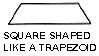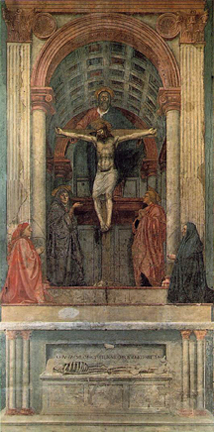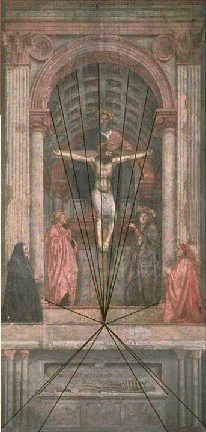Masaccio, Trinity, 1427-28 Fresco, 21' 10 1/2" x 10' 4 7/8" (6.67 x 3.17 m) Santa Maria Novella, Florence Paolo Uccello, The Rout of San Romano, c. 1456 (National Gallery, London) Leonardo da Vinci, The Adoration of the Magi, 1481-1482 Yellow ochre and brown ink on panel 246 x 243 cm (8 x 8 ft.) (Uffizi, Florence) Leonardo da Vinci, perspective drawing for The Adoration of the Magi